# 技术干货 | 基于 MindSpore 更好的理解 Focal Loss​​​​​​​​​​​​​​摘要：FocalLoss，是 Kaiming 大神团队在他们的论文 Focal Loss for Dense Object Detection 提出来的损失函数，利用它改善了图像物体检测的效果。

• 使用场景

1. 设计采样策略，一般都是对数量少的样本进行重采样

2. 设计 Loss，一般都是对不同类别样本进行权重赋值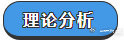## 论文分析

So，Why？and result？

(1)training is inefficient as most locations are easy negatives that contribute nouseful learning signal;

(2) en masse, the easy negatives can overwhelm training and lead to degeneratemodels.

## 公式说明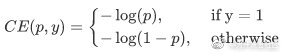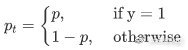​为了表示简便，我们用 p_t 表示样本属于 true class 的概率。所以(1)式可以写成: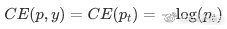​接下来介绍一个最基本的对交叉熵的改进，也将作为本文实验的 baseline，既然 one-stage detector 在训练的时候正负样本的数量差距很大，那么一种常见的做法就是给正负样本加上权重，负样本出现的频次多，那么就降低负样本的权重，正样本数量少，就相对提高正样本的权重。因此可以通过设定α的值来控制正负样本对总的 loss 的共享权重。

α取比较小的值来降低负样本（多的那类样本）的权重。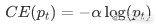​显然前面的公式 3 虽然可以控制正负样本的权重，但是没法控制容易分类和难分类样本的权重，于是就有了 FocalLoss，这里的γ称作 focusing parameter，γ>=0，称为调制系数：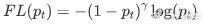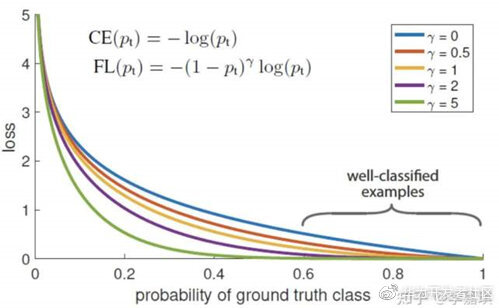​这样就既做到了解决正负样本不平衡，也做到了解决 easy 与 hard 样本不平衡的问题。

## 结论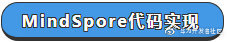import mindsporeimport mindspore.common.dtype as mstypefrom mindspore.common.tensor import Tensorfrom mindspore.common.parameter import Parameterfrom mindspore.ops import operations as Pfrom mindspore.ops import functional as Ffrom mindspore import nnclass FocalLoss(_Loss):    def __init__(self, weight=None, gamma=2.0, reduction='mean'):        super(FocalLoss, self).__init__(reduction=reduction)        # 校验gamma，这里的γ称作focusing parameter，γ>=0，称为调制系数        self.gamma = validator.check_value_type("gamma", gamma, [float])        if weight is not None and not isinstance(weight, Tensor):            raise TypeError("The type of weight should be Tensor, but got {}.".format(type(weight)))        self.weight = weight        # 用到的mindspore算子        self.expand_dims = P.ExpandDims()        self.gather_d = P.GatherD()        self.squeeze = P.Squeeze(axis=1)        self.tile = P.Tile()        self.cast = P.Cast()    def construct(self, predict, target):        targets = target        # 对输入进行校验        _check_ndim(predict.ndim, targets.ndim)        _check_channel_and_shape(targets.shape, predict.shape)        _check_predict_channel(predict.shape)        # 将logits和target的形状更改为num_batch * num_class * num_voxels.        if predict.ndim > 2:            predict = predict.view(predict.shape, predict.shape, -1) # N,C,H,W => N,C,H*W            targets = targets.view(targets.shape, targets.shape, -1) # N,1,H,W => N,1,H*W or N,C,H*W        else:            predict = self.expand_dims(predict, 2) # N,C => N,C,1            targets = self.expand_dims(targets, 2) # N,1 => N,1,1 or N,C,1                # 计算对数概率        log_probability = nn.LogSoftmax(1)(predict)        # 只保留每个voxel的地面真值类的对数概率值。        if target.shape == 1:            log_probability = self.gather_d(log_probability, 1, self.cast(targets, mindspore.int32))            log_probability = self.squeeze(log_probability)        # 得到概率        probability = F.exp(log_probability)        if self.weight is not None:            convert_weight = self.weight[None, :, None]  # C => 1,C,1            convert_weight = self.tile(convert_weight, (targets.shape, 1, targets.shape)) # 1,C,1 => N,C,H*W            if target.shape == 1:                convert_weight = self.gather_d(convert_weight, 1, self.cast(targets, mindspore.int32))  # selection of the weights  => N,1,H*W                convert_weight = self.squeeze(convert_weight)  # N,1,H*W => N,H*W            # 将对数概率乘以它们的权重            probability = log_probability * convert_weight        # 计算损失小批量        weight = F.pows(-probability + 1.0, self.gamma)        if target.shape == 1:            loss = (-weight * log_probability).mean(axis=1)  # N        else:            loss = (-weight * targets * log_probability).mean(axis=-1)  # N,C        return self.get_loss(loss)

from mindspore.common import dtype as mstypefrom mindspore import nnfrom mindspore import Tensorpredict = Tensor([[0.8, 1.4], [0.5, 0.9], [1.2, 0.9]], mstype.float32)target = Tensor([, , ], mstype.int32)focalloss = nn.FocalLoss(weight=Tensor([1, 2]), gamma=2.0, reduction='mean')output = focalloss(predict, target)print(output)0.33365273

Focal Loss 的两个重要性质

1. 当一个样本被分错的时候，pt 是很小的，那么调制因子（1-Pt）接近 1，损失不被影响；当 Pt→1，因子（1-Pt）接近 0，那么分的比较好的（well-classified）样本的权值就被调低了。因此调制系数就趋于 1，也就是说相比原来的 loss 是没有什么大的改变的。当 pt 趋于 1 的时候（此时分类正确而且是易分类样本），调制系数趋于 0，也就是对于总的 loss 的贡献很小。

2.当γ=0 的时候，focal loss 就是传统的交叉熵损失，当γ增加的时候，调制系数也会增加。 专注参数γ平滑地调节了易分样本调低权值的比例。γ增大能增强调制因子的影响，实验发现γ取 2 最好。直觉上来说，调制因子减少了易分样本的损失贡献，拓宽了样例接收到低损失的范围。当γ一定的时候，比如等于 2，一样 easyexample(pt=0.9)的 loss 要比标准的交叉熵 loss 小 100+倍，当 pt=0.968 时，要小 1000+倍，但是对于 hard example(pt < 0.5)，loss 最多小了 4 倍。这样的话 hard example 的权重相对就提升了很多。这样就增加了那些误分类的重要性。

Focal Loss 的两个性质算是核心，其实就是用一个合适的函数去度量难分类和易分类样本对总的损失的贡献。MindSpore 官方资料 GitHub : https://github.com/mindspore-ai/mindspore## 评论